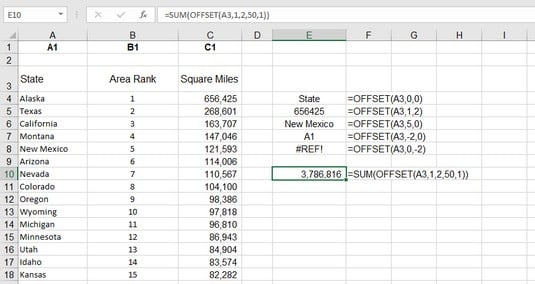##### Excel Formulas & Functions For DummiesExcel’s OFFSET function lets you get the address of the cell that is offset from another cell by a certain number of rows and/or columns. For example, cell E4 is offset from cell B4 by three columns because it is three columns to the right. The Excel OFFSET takes up to five arguments. The first three are required:
• A cell address or a range address: Named ranges are not allowed.
• The number of rows to offset: This can be a positive or negative number. Use 0 for no row offset.
• The number of columns to offset: This can be a positive or negative number. Use 0 for no column offset.
• The number of rows in the returned range: The default is the number of rows in the reference range (the first argument).
• The number of columns to return: The default is the number of columns in the reference range.
If you omit the last two arguments, OFFSET returns a reference to a single cell. If you include a value greater than 1 for either or both, the function's return references a range of the specified size with the top-left cell at the specified offset.

The following image shows some examples of using Excel’s OFFSET function. Columns A through C contain a ranking of the states in the United States by size in square miles. Column E shows how OFFSET has returned different values from cells that are offset from cell A3.Finding values by using the Excel OFFSET function.

Some highlights follow:

• Cell E4 returns the value of cell A3 because both the row and column offset is set to 0: `=OFFSET(A3,0,0)`.
• Cell E7 returns the value you find in cell A1 (the value also is `A1`). This is because the row offset is –2. From the perspective of A3, minus two rows is row number 1: `=OFFSET(A3,-2,0)`.
• Cell E8 displays an error because OFFSET is attempting to reference a column that is less than the first column: `=OFFSET(A3,0,-2)`.
• Cell E10 makes use of the two optional OFFSET arguments to tell the SUM function to calculate the sum of the range C4:C53: `=SUM(OFFSET(A3,1,2,50,1))`.
Here's how to use the OFFSET function:
1. Click a cell where you want the result to appear.
2. Type =OFFSET( to start the function.
3. Enter a cell address or click a cell to get its address.
4. Type a comma (,).
5. Enter the number of rows you want to offset where the function looks for a value.

This number can be a positive number, a negative number, or 0 for no offset.

6. Type a comma (,).
7. Enter the number of columns you want to offset where the function looks for a value.

This can be a positive number, a negative number, or 0 for no offset.

8. Type a ) and press Enter.
OFFSET is another of those functions that can be used alone but is usually used as part of a more complex formula.